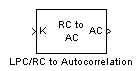# LPC/RC to Autocorrelation

Convert linear prediction coefficients or reflection coefficients to autocorrelation coefficients

## Library

Estimation / Linear Prediction

`dsplp`

•## Description

The LPC/RC to Autocorrelation block either converts linear prediction coefficients (LPCs) to autocorrelation coefficients (ACs) or reflection coefficients (RCs) to autocorrelation coefficients (ACs). Set the Type of conversion parameter to `LPC to autocorrelation` or ```RC to autocorrelation``` to select the domain from which you want to convert your coefficients. The A port corresponds to LPC coefficients, and the K port corresponds to the RC coefficients.

The block input can be an N-by-M matrix or an unoriented vector. Each column of the matrix is treated as a channel. When the input is an unoriented vector, the input is treated as one channel.

Use the Specify P parameter to set the value of the prediction error power. You can set this parameter to 1 by selecting ```Assume P=1```. When you select `Via input port`, a P port appears on the block. You can use this port to input the value of the actual, non-unity prediction error power for each channel. The length of this vector must equal the number of channels in the input.

The If first input value is not 1 parameter specifies the behavior of the block when the first coefficient of the LPC coefficient vector is not 1. The following options are available:

• `Replace it with 1` — The block changes the first value of the coefficient vector to 1. The rest of the coefficient values are unchanged.

• `Normalize` — The block divides the entire vector of coefficients by the first coefficient so that the first coefficient of the LPC coefficient vector is 1.

• `Normalize and Warn` — The block divides the entire vector of coefficients by the first coefficient so that the first coefficient of the LPC coefficient vector is 1. The block displays a warning message telling you that your vector of coefficients has been normalized.

• `Error` — The block displays an error telling you that the first coefficient of the LPC coefficient vector is not 1.

## Parameters

Type of conversion

From the list select `LPC to autocorrelation `or `RC to autocorrelation` to specify the domain from which you want to convert your coefficients.

Specify P

From the list select `Assume P=1` or `Via input port` to specify the value of prediction error power.

If first input value is not 1

Select what you would like the block to do when the first coefficient of the LPC coefficient vector is not 1. You can choose ```Replace it with 1```, `Normalize`, `Normalize and Warn`, and `Error`.

## References

Orfanidis, S.J. Optimum Signal Processing. New York, McGraw-Hill, 1988.

Makhoul, J. Linear Prediction: A tutorial review. Proc. IEEE. 63, 63, 56 (1975).

Markel, J.D. and A. H. Gray, Jr., Linear Prediction of Speech. New York, Springer-Verlag, 1976.

## Supported Data Types

• Double-precision floating point

• Single-precision floating point